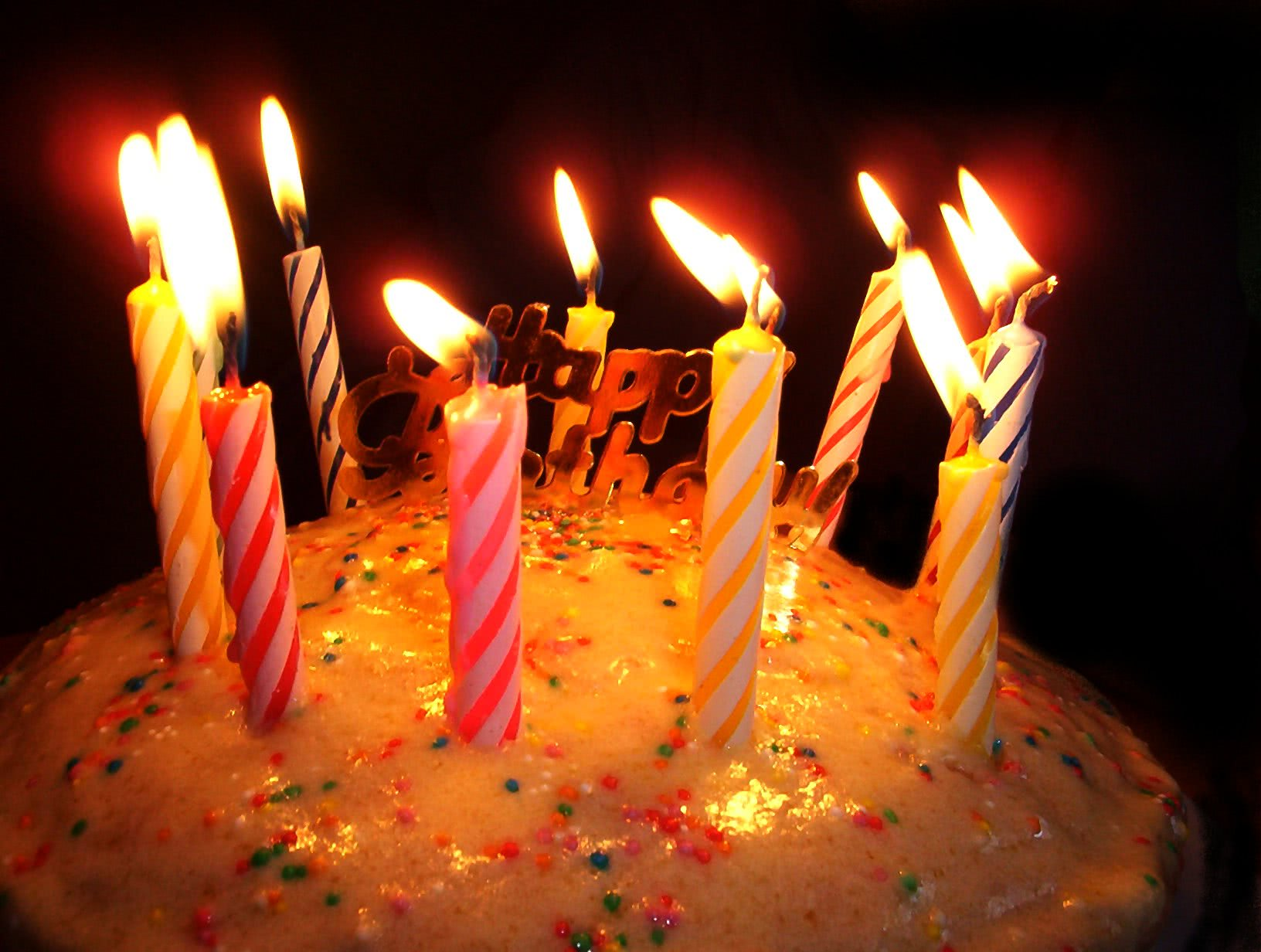## The birthday bet

In high school I had a colleague that had his birthday on the same day as I did. What a coincidence, right? Right..?This post has the purpose of presenting a result that may seem counterintuitive and that can provide a really nice excuse for a wager between you and one or more of your friends. For this post, when I talk about a birthdate I am only referring to the day and month of birth, not the year.

What is the probability that you and your best friend have the same birth day and month? Even without an exact number one knows that you are much more likely to have different birthdates than having equal birthdates. Assuming all $$366$$ days are equally likely, the probability that two people have the same birthdate is $$\frac{1}{366} \approx 0.27\%$$ and the probability that the birthdate is different is $$\frac{365}{366} \approx 99.73\%$$.

What would your guess be? Half of 366, 183 people? 100? 50? 10?

It only takes $$23$$ people! If you have a group of $$23$$ people, the probability that no one shares the birthdate is approximately $$49.37\%$$! That is the same as saying that, in a group of $$23$$ people, there is a $$\approx 50.63\%$$ chance that two people share a birthdate.

This seems very counterintuitive because $$23$$ people can only cover $$23$$ of the $$366$$ possible days, which represents a ratio of $$\frac{23}{366}\approx 6.3\%$$, a very low number which wouldn't make us think that $$23$$ people were enough to make this happen. But why would you care?

Whenever you find yourself in a group of $$23$$ people or more, you can bet the other people of the group that at least two of you share the same birthdate. If you do this often enough, you will make money! Just like a casino: you will win some and lose some, but in the long run you are expected to profit from this.

• In a group of $$23$$, your chances of winning are above $$50\%$$;
• In a group of $$27$$, your chances of winning are above $$60\%$$;
• In a group of $$30$$, your chances of winning are above $$70\%$$;
• In a group of $$35$$, your chances of winning are above $$80\%$$;
• In a group of $$41$$, your chances of winning are above $$90\%$$.

How can you compute these probabilities? Take the $$n$$ people of your group and line them up. We will be comparing the birthdate of a person with the birthdates of everyone to the left. What is the probability that the second person has a birthdate distinct from the first person? It is $$\frac{365}{366}$$. What is the probability that the third person has a birthdate distinct from the two people to the left, if the two to the left have distinct birthdates? It is $$\frac{364}{366}$$, so what is the probability that the first three people have distinct birthdates? It is $$\frac{365}{366}\times\frac{364}{366}$$, the probability that the second person doesn't share its birthdate with the first person times the probability that the third person doesn't share its birthdate with the first or second people.

We can keep this train of thought going: if the first three people have different birthdates, what is the probability that the fourth person has a distinct birthdate? It is $$\frac{363}{366}$$, so the probability that the first four people have different birthdates is $$\frac{365}{366}\times\frac{364}{366}\times\frac{363}{366}$$.

In a general setting, the probability that $$n < 366$$ people have distinct birthdates is

$\prod_{i=1}^{n-1} \frac{366-i}{366}$

The text field above will allow you to compute the probability of two people sharing birthdates in a group with a given number of people. Writing $$31$$ and hitting the button allows one to conclude that in my classroom of $$31$$ students, there was a $$72.95\%$$ chance of two people sharing a birthdate... In high school, it ended up being me sharing my birthdate with Gustavo!